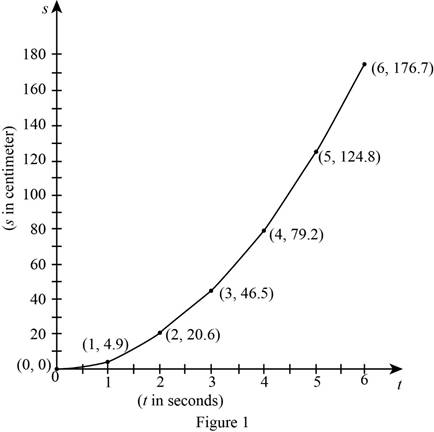# The average velocity for given time periods.### Single Variable Calculus: Concepts...

4th Edition
James Stewart
Publisher: Cengage Learning
ISBN: 9781337687805### Single Variable Calculus: Concepts...

4th Edition
James Stewart
Publisher: Cengage Learning
ISBN: 9781337687805

#### Solutions

Chapter 2.1, Problem 7E

(a)

To determine

## To find: The average velocity for given time periods.

Expert Solution

(i) The average velocity over the time interval [2, 4] is 29.3 ft/s.

(ii) The average velocity over the time interval [3, 4] is 32.7 ft/s.

(iii) The average velocity over the time interval [4, 5] is 45.6 ft/s.

(iv) The average velocity over the time interval [4, 6] is 48.75 ft/s.

### Explanation of Solution

Formula used:

The average velocity over the time interval [t,t+h] is,

vavg=s(t+h)s(t)(t+h)t (1)

Calculation:

Section-(i)

Obtain the average velocity over the time interval [2, 4].

Substitute t=2 and t+h=4 in equation (1),

vavg=s(t+h)s(t)(t+h)t=s(4)s(2)42=s(4)s(2)2

From the given table, it is observed that,

(i) When t=2 seconds, the value of s(2)=20.6 ft.

(ii) When t=4 seconds, the value of s(4)=79.2 ft.

Thus, the average velocity over the time interval [2, 4] is computed as follows.

vavg=s(4)s(2)2=79.220.62=58.62=29.3

Therefore, the average velocity over the time interval [2, 4] is 29.3 ft/s.

Section-(ii)

Obtain the average velocity over the time interval [3, 4].

Substitute t=3 and t+h=4 in equation (1),

vavg=s(t+h)s(t)(t+h)t=s(4)s(3)43=s(4)s(3)1

From the given table, it is observed that,

(i) When t=3 seconds, the value of s(3)=46.5 ft.

(ii) When t=4 seconds, the value of s(4)=79.2.

Thus, the average velocity over the time interval [3, 4] is computed as follows.

vavg=s(4)s(3)=79.246.5=32.7

Therefore, the average velocity over the time interval [3, 4] is 32.7 ft/s.

Section-(iii)

Obtain the average velocity over the time interval [4, 5].

Substitute t=4 and t+h=5 in equation (1),

vavg=s(t+h)s(t)(t+h)t=s(5)s(4)54=s(5)s(4)1

From the table, it is observed that,

(i) When t=4 seconds, the value of s(4)=79.2 ft.

(ii) When t=5 seconds, the value of s(5)=124.8.

Thus, the average velocity over the time interval [4, 5] is computed as follows.

vavg=s(5)s(4)=124.879.2=45.6

Therefore, the average velocity over the time interval [4, 5] is 45.6 ft/s.

Section-(iv)

Obtain the average velocity over the time interval [4, 6].

Substitute t=4 and t+h=6 in equation (1),

vavg=s(t+h)s(t)(t+h)t=s(6)s(4)64=s(6)s(4)2

From the table, it is observed that,

(i) When t=4 seconds, the value of s(4)=79.2 ft.

(ii) When t=6 seconds, the value of s(6)=176.7.

Thus, the average velocity over the time interval [4, 6] is computed as follows.

vavg=s(6)s(4)2=176.779.22=97.52=48.75

Therefore, average velocity over the time interval [4, 6] is 48.75 ft/s .

(b)

To determine

### To estimate: The instantaneous velocity when t=3.

Expert Solution

The estimated instantaneous velocity when t=3 is 29.67 ft/s.

### Explanation of Solution

Plot a curve using the points (0, 0), (1, 4.9), (2, 20.6), (3, 46.5), (4, 79.2), (5, 124.8) and (6, 176.7) as shown below in Figure 1.Draw the slope of the tangent line at t=3 seconds as shown below in Figure 2.The instantaneous velocity at t=3 is equal to the slope of the tangent line at (3, 46.5).

From Figure 2, the slope of the tangent line at (3, 46.5) is obtained below.

mΔRSΔST105165289329.67

The estimated instantaneous velocity when t=3 is 29.67 ft/s.

### Have a homework question?

Subscribe to bartleby learn! Ask subject matter experts 30 homework questions each month. Plus, you’ll have access to millions of step-by-step textbook answers!